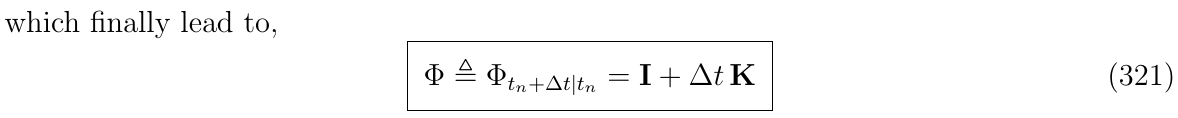# 2. 龙格库塔数值积分法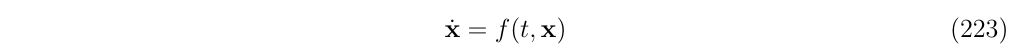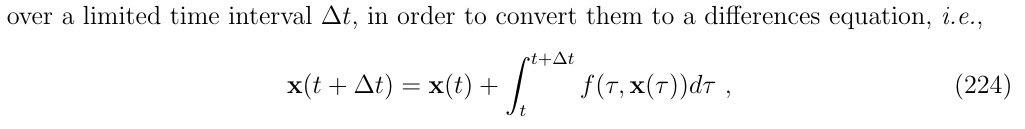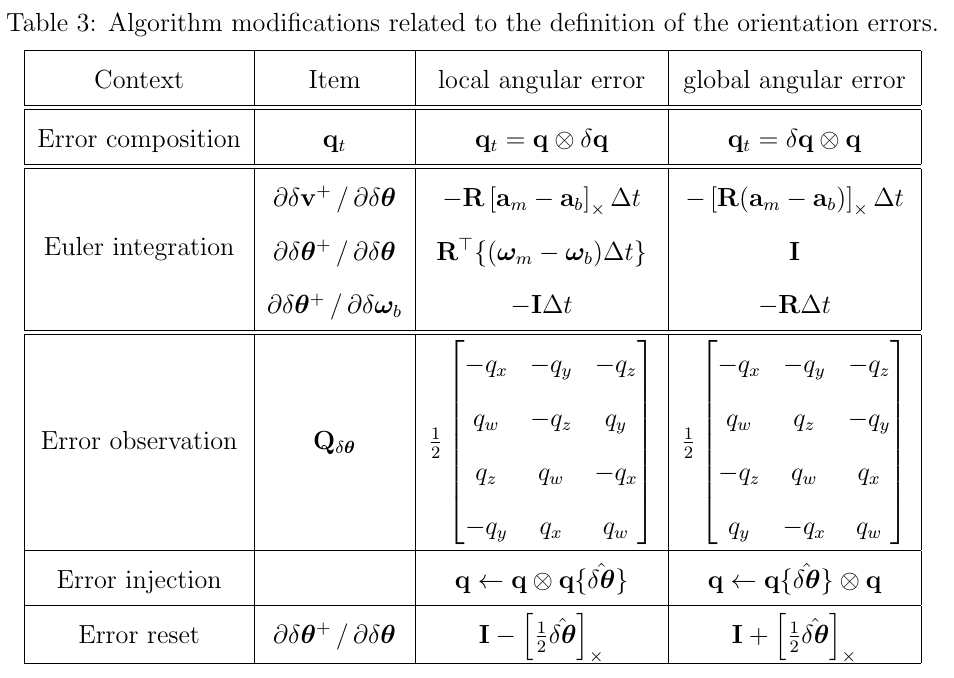## 2.1. 欧拉方法(The Euler method)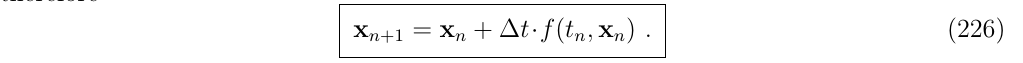1. 计算在初始点处的导数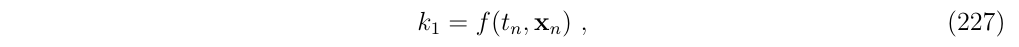1. 使用它来计算积分，到结束点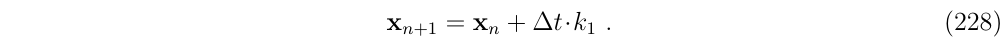## 2.2. 中点法(The midpoint method)1. 使用欧拉方法积分，直到中点 (使用前面定义的k1)1. 然后使用这个值来计算中间点k2的导数，然后对后半段进行积分## 2.3. 4阶龙格库塔法(The RK4 method)

RK4方法比上面两种方法的更好，其积分计算步骤是:• k1是区间开始时的斜率，使用$$x_n$$来计算. (欧拉方法)
• k2是区间中点处的斜率，使用$$x_n+\frac{1}{2}\Delta t k_1$$来计算。(中点法)
• k3还是中点的斜率，不过使用$$x_n+\frac{1}{2}\Delta t k_2$$计算
• k4是区间最后的斜率，使用$$x_n+\Delta t k_3$$计算

## 2.4. 通用龙格库塔法(General Runge-Kutta method)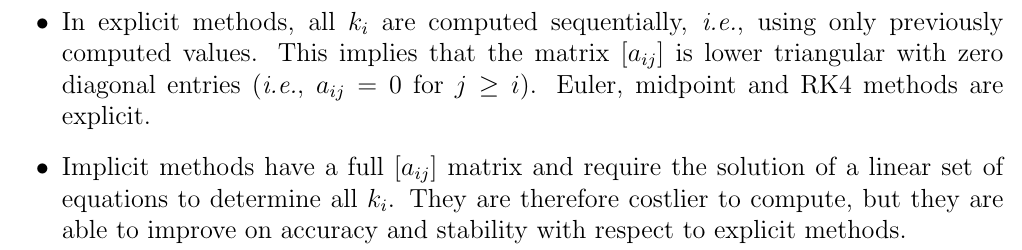# 3. 闭式积分方法(Closed-form integration methods)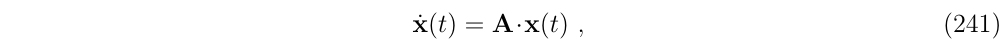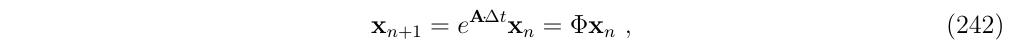## 3.1. 角度误差的积分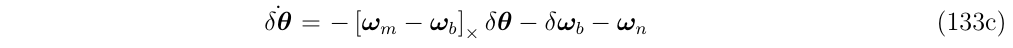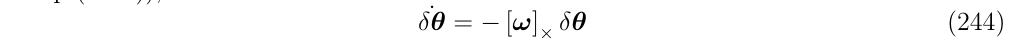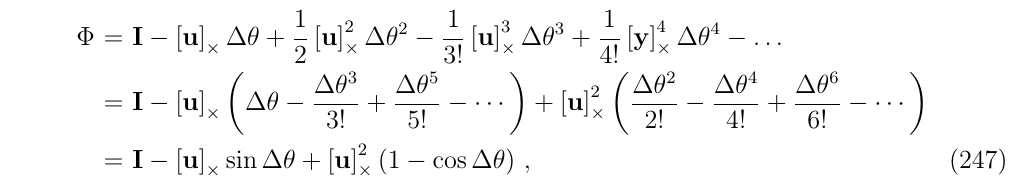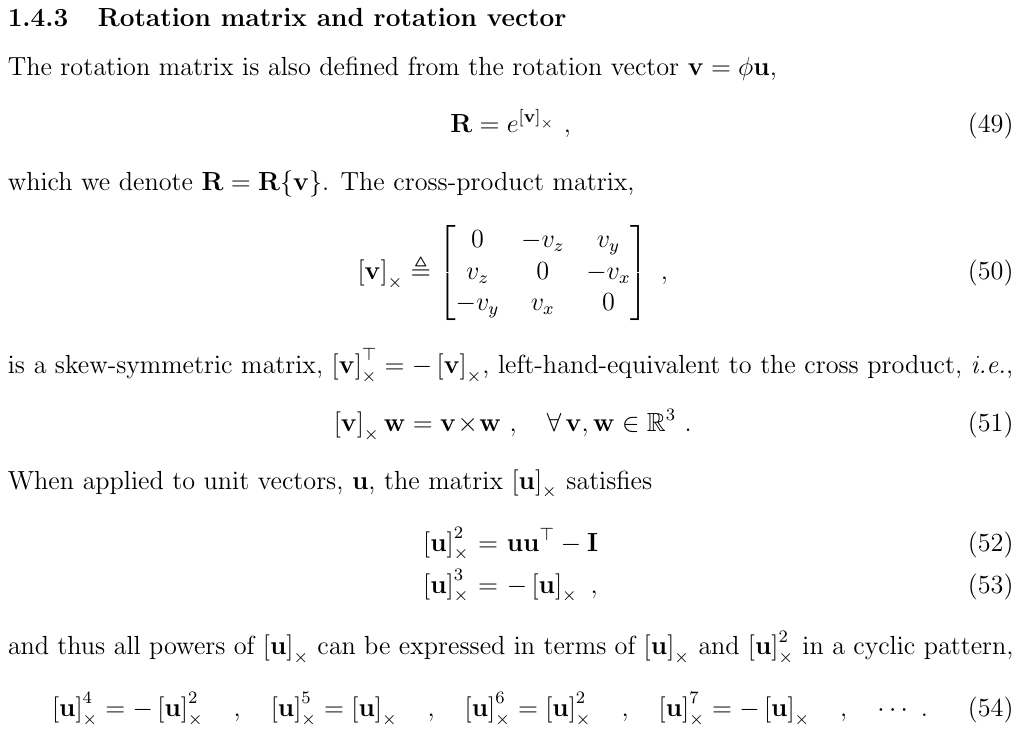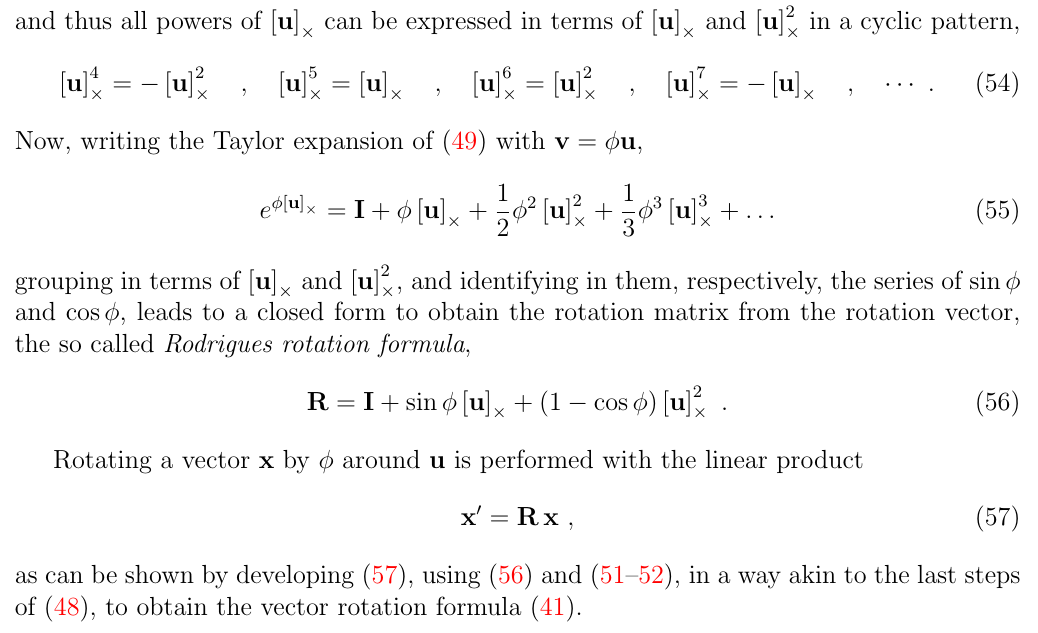$\Phi=R\{-\boldsymbol{u} \Delta \theta \}=R\{-\boldsymbol{w} \Delta t\}=R\{\boldsymbol{w} \Delta t\}^T$## 3.2. 简化版IMU例子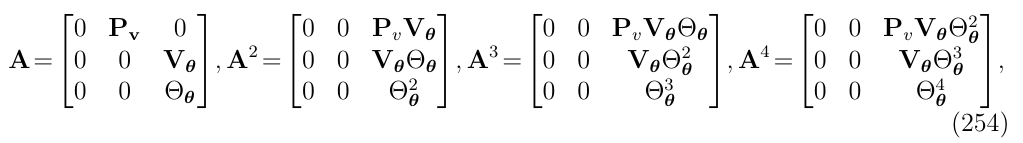1. Trivial diagonal terms (对角线前2个)

1. Rotational diagonal term (对角线第3项)1. Position-vs-velocity term (第一行第二列)1. Velocity-vs-angle term (第二行第三列)(1)我们可以把级数的第一个有效项截断，即只保留$$\Phi_{v,\theta}=V_{\theta}\Delta t$$ ，但是这样就不是闭式解了。(有关使用这种简化方法的结果，请参见下一节)

(2)第二种方法，把因式$$V_{\theta}$$提取出来，如下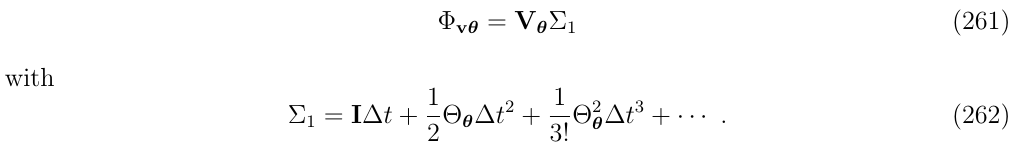• $$\Sigma_1$$中的$$\Theta_\theta$$次方项与系数$$\frac{1}{k!}$$以及$$\Delta t$$次方项都不匹配。实际上，我们在这里$$\Sigma_1$$的标记1表明$$\Theta_\theta$$次方项少了1次
• 还有一个问题是，在这个多项式的开头，少了一些内容。实际上，在这里$$\Sigma_1$$的标记1表明少了1些项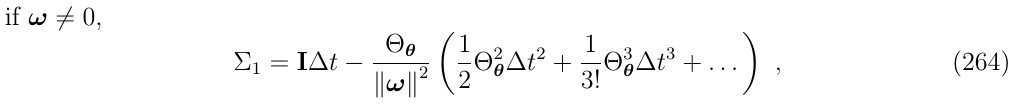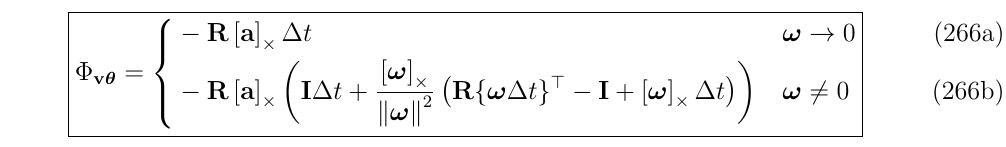1. Position-vs-angle term (第一行第三列)

$$\Phi$$矩阵的第一行第三列$$\Phi_{p\theta}$$展开:• 对于上面多项式的每一项，都少了$$\Theta_\theta$$的2次
• 对于上面的多项式，缺少了开头两项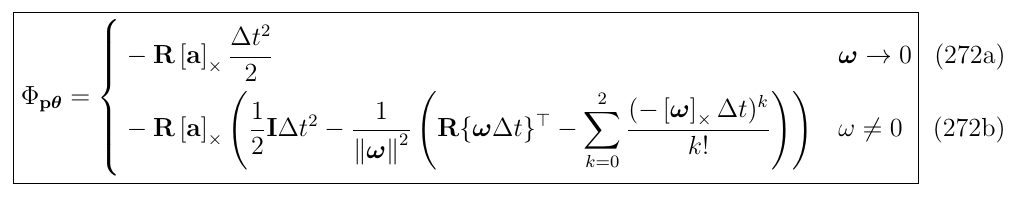## 3.3. 完整版IMU例子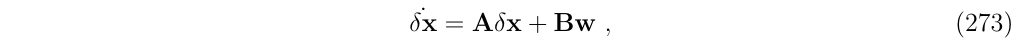• $$P_v=I$$
• $$V_\theta=-R[a_m-a_b]_\times$$
• $$V_a=-R$$
• $$V_g=I$$
• $$\Theta_{\theta}=-[w_m-w_b]_\times$$
• $$\Theta_w=-I$$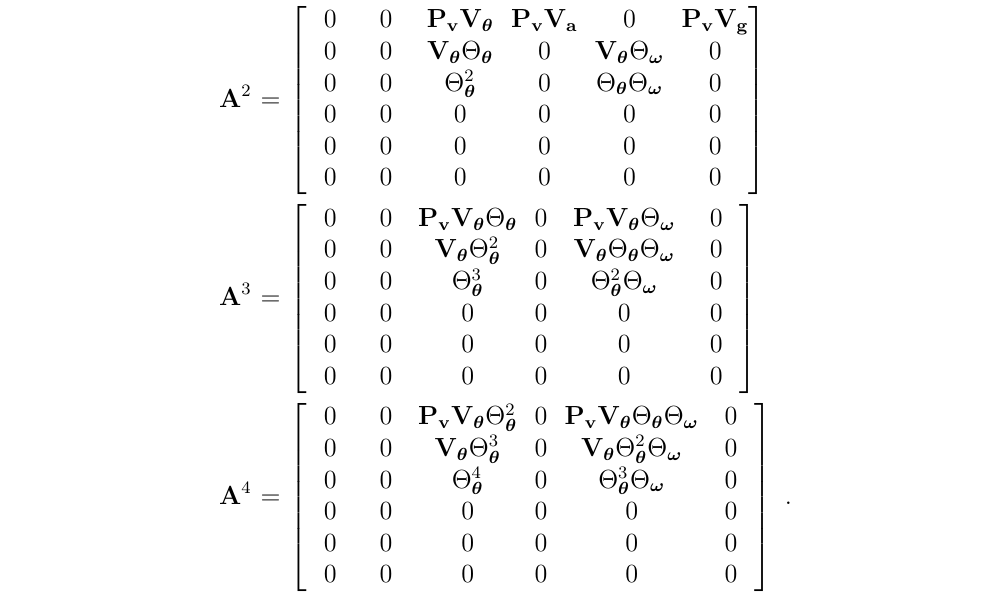• A的对角线上的唯一一项，旋转项$$\Theta_\theta$$，在$$A^k$$的次方序列中向右和向上传播。所有不受传播影响的项都将消失，这个传播在以下3个方面影响了$$\{A^k \}$$序列的结构:
• 三次幂后，A的稀疏性趋于稳定，也就是说，对于大于3的幂，不再出现或消失非零块
• 左上角3x3的子块，与前面例子中的简化IMU模型相对应，在这个例子里面仍然没有改变，因此，这个子块的闭式解就是前面得到的结果。
• 与角速度偏置(gyrometer bias)误差的相关项(在第5列中的项)，产生了与$$\Theta_\theta$$次方序列相似的项，可以采用上面“简化版IMU”所使用的技巧进行求解。

• 下标表示了序列中每个元素缺失的$$\Theta_\theta$$的次方数
• 下标表示了序列开头的项的数目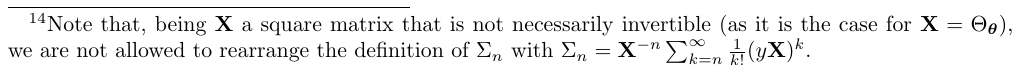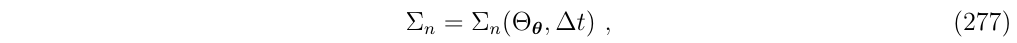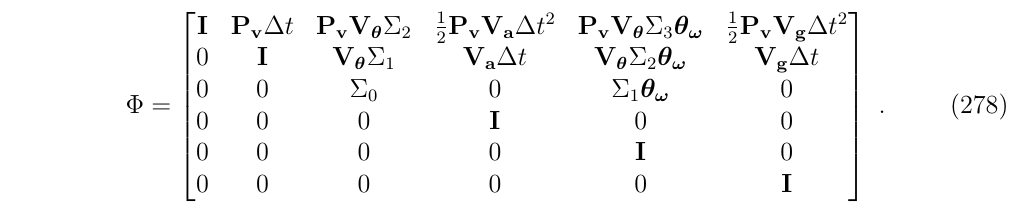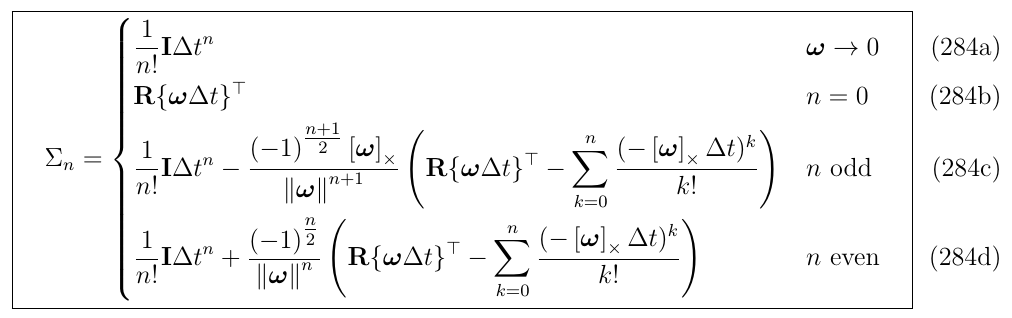# 4. 使用截断序列的近似方法(重点)

## 4.1. system-wise截断

### 4.1.1. 一阶截断: 有限差分法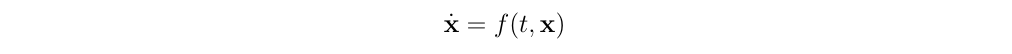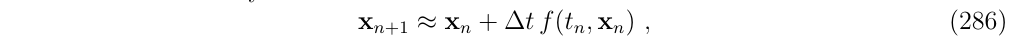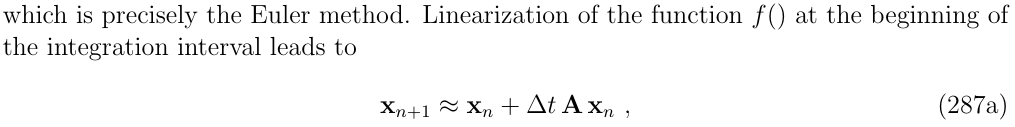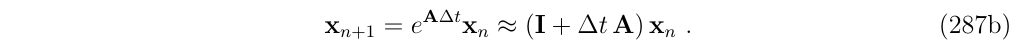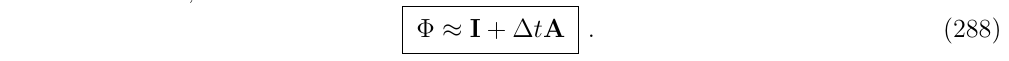### 4.1.2. N阶截断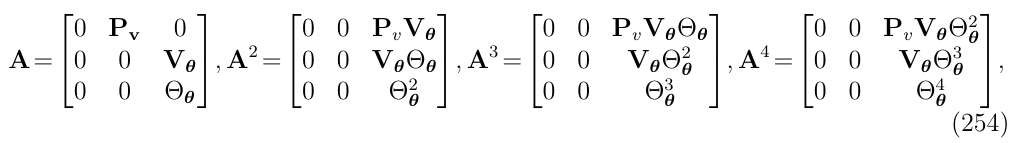## 4.2. Block-wise截断(这个好用)

### 4.2.1. 简化版IMU例子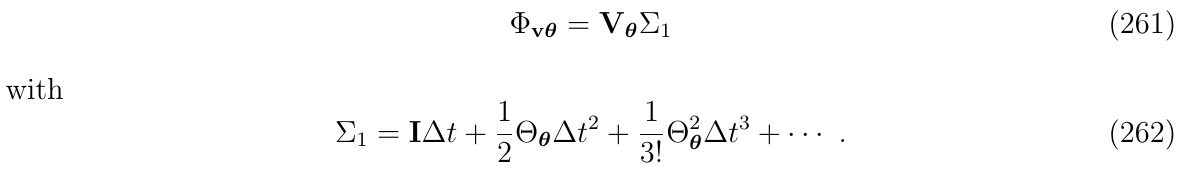### 4.2.2. 完整版IMU例子

(1)标准的状态转移矩阵$$\Phi$$:• $$P_v=I$$
• $$V_\theta=-R[a_m-a_b]_\times$$
• $$V_a=-R$$
• $$V_g=I$$
• $$\Theta_{\theta}=-[w_m-w_b]_\times$$
• $$\Theta_w=-I$$

(2)$$\Sigma_n$$的闭式表达:(3)根据上面的推导进行截断，得到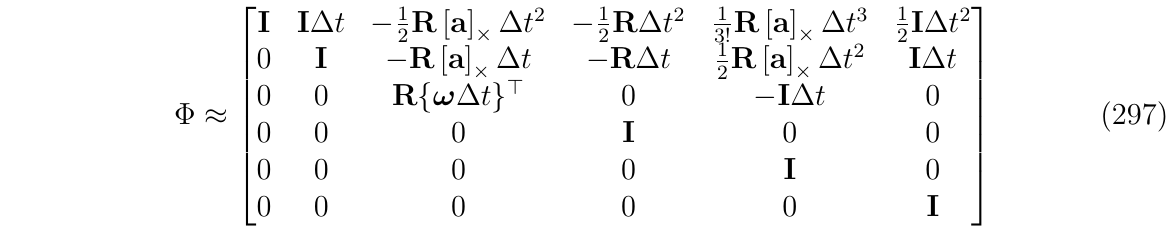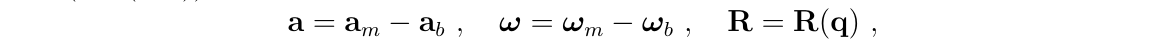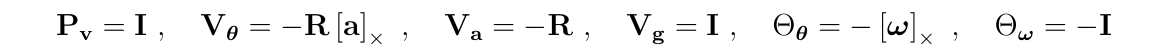(4)如果还想要进一步简化，一个直接的方法是限制每个子块的最高阶数n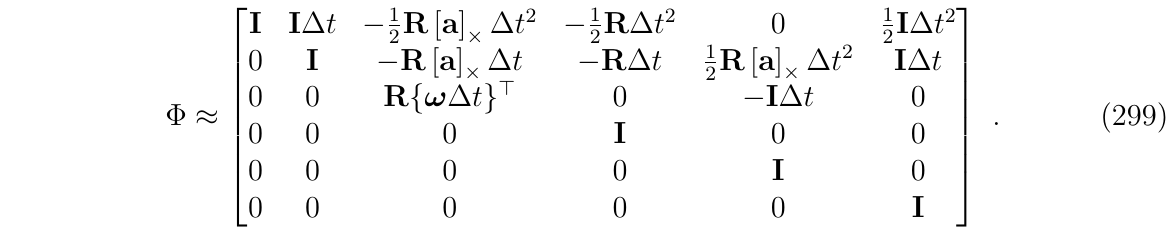# 5. 使用龙格库塔积分法得到状态转移矩阵(时变)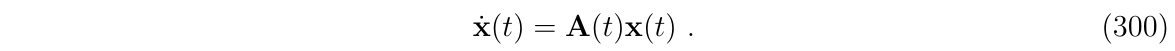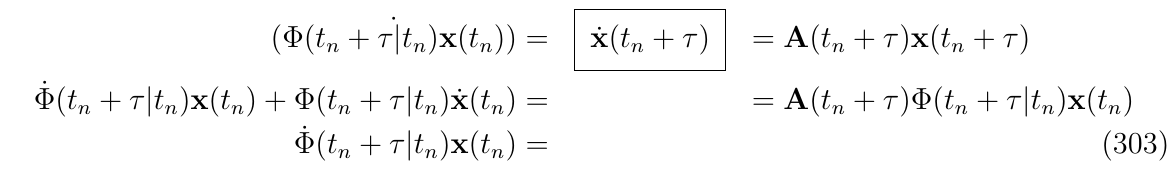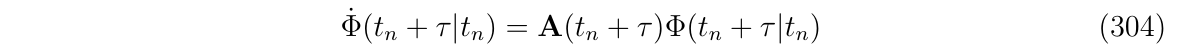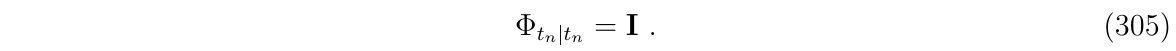## 5.1. 举例: 误差状态方程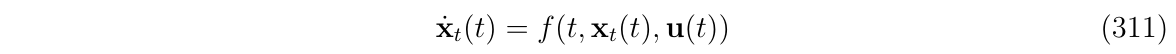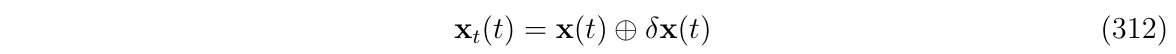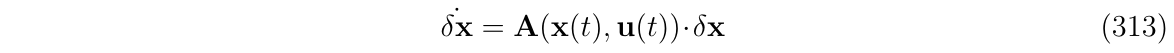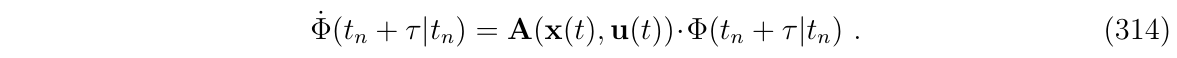Nominal state方程应采用最优的方法进行积分，例如，使用RK4: